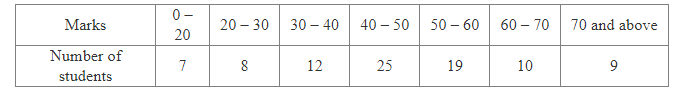# The marks obtained by 90 students of a school in mathematics out of 100 are given as under:

Question:

The marks obtained by 90 students of a school in mathematics out of 100 are given as under:From these students, a student is chosen at random.
What is the probability that the chosen student
(i) gets 20% or less marks?
(ii) gets 60% or more marks?

Solution:

Total number of students = 90

(i) Number of students who gets 20% or less marks = Number of students who gets 20 or less marks = 7

$\therefore \mathrm{P}($ Student gets $20 \%$ or less marks $)=\frac{\text { Number of students who gets } 20 \% \text { or less marks }}{\text { Total number of students }}=\frac{7}{90}$

(ii) Number of students who gets 60% or more marks = Number of students who gets 60 or more marks = 10 + 9 = 19

$\therefore \mathrm{P}($ Student gets $60 \%$ or more marks $)=\frac{\text { Number of students who gets } 60 \% \text { or more marks }}{\text { Total number of students }}=\frac{19}{90}$